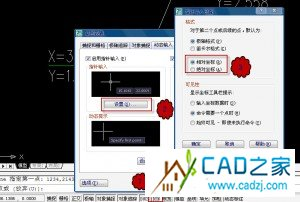1. x=2134 y=1234
2. x=3142 y=1324

1. x=2134 y=1234
2. x=5276 y=2558